Categories :

## MATH SAMPLE PAPER TEST FOR BOARD EXAM 2023

CBSE Maths Sample Test Paper for Class X: KV Maths Sample Paper Class 10 2023 is now available for practice. The Central Board of Secondary education has released CBSE Class 10 Sample Question Paper 2023 for all subjects. The CBSE will conduct the Class 10 board exams for the academic year 2022-23 from February 15 onwards.

SUBJECT: MATHEMATICS CLASS: X MAX. MARKS: 80 DURATION : 3 HRS

General Instruction:
1. This Question Paper has 5 Sections A-E.
2. Section A has 20 MCQs carrying 1 mark each.
3. Section B has 5 questions carrying 02 marks each.
4. Section C has 6 questions carrying 03 marks each.
5. Section D has 4 questions carrying 05 marks each.
6. Section E has 3 case based integrated units of assessment (04 marks each) with sub-parts of the
values of 1, 1, and 2 marks each respectively.
7. All Questions are compulsory. However, an internal choice in 2 Qs of 5 marks, 2 Qs of 3 marks, and 2 Questions of 2 marks has been provided.
8. Draw neat figures wherever required. Take π =22/7 wherever required if not stated.

### Maths Sample Paper for Class X – Board Exam 2023

SECTION – A Questions 1 to 20 carry 1 mark each.

1. The LCM of the two numbers is 14 times their HCF. The sum of LCM and HCF is 600. If one number is 280, then the other number is

(a) 20 (b) 28 (c) 60 (d) 80

2. When 2120 is expressed as the product of its prime factors we get

(a) 2 × 53 × 53 (b) 23 × 5 × 53 (c) 5 × 72 × 31 (d) 52 × 7 × 33

3. If p and g are the zeroes of the quadratic polynomial f(x) = 2×2 – 7x + 3, find the value of p + g – PQ is

(a) 1 (b) 2 (c) 3 (d) None of these

4. The pair of linear equations 2x + 3y = 5 and 4x + 6y = 10 is

(a) inconsistent (b) consistent (c) dependent consistent (d) none of these

5. Points A(3, 1), B(5, 1), C(a, b) and D(4, 3) are vertices of a parallelogram ABCD. The values of a and b are respectively

(a) a = 6, b = 3 (b) a = 2, b = 1 (c) a = 4, b = 2 (d) None of these

6. IfAABC ~ ADEF, BC = 3 cm, EF = 4 cm and area of AABC = 54 cm?. Then the area of ADEF is

(a) 54 cm² (b) 88 cm² (c) 96 cm² (d) 108 cm²

7. If sec A = 15/7 and A + B = 90°, find the value of cosec B.

(a) 8/7 (b) 12/7 (c) 7/15 (d) 15/7

8. In AABC, right angled at B, AB = 5 cm and sin C = 1/2. Determine the length of the side AC.

(a) 10 cm (b) 15 cm (c) 20 cm (d) none of these

9. In the AABC, D, and E are points on side AB and AC respectively such that DE || BC. If AE = 2
cm, AD = 3 cm and BD = 4.5 cm, then CE equals

(a) 1 cm (b) 2 cm (c) 3 cm (d) 4 cm

10. ABCD is a trapezium with AD I| BC and AD = 4cm. If the diagonals AC and BD intersect each
other at O such that AO/OC = DO/OB =1/2, then BC =

(a) 6cm (b) 7cm (c) 8cm (d) 9cm

11. If the angle between two radii of a circle is 140°, then the angle between the tangents at the ends

(a) 90° (b) 50° (c) 70° (d) 40°

12. The number of revolutions made by a circular wheel of radius 0.7 m in rolling a distance of 176 m is

(a) 22 (b) 24 (c) 75 (d) 40

13. The area of the square that can be inscribed in a circle of radius 8 cm is

(a) 256 cm² (b) 128 cm² (c) 64√2 cm² (d) 64 cm²

14. The ratio of the total surface area to the lateral surface area of a cylinder with a base radius of 80 cm and height of 20 cm is

(a) 1: 2 (b) 2: 1 (c) 3: 1 (d) 5: 1

15. The mean and mode of a frequency distribution are 28 and 16 respectively. The median is

(a) 22 (b) 23.5 (c) 24 (d) 24.5

16. The median class of the following data is:

17. Two dice are thrown simultaneously. What is the probability of getting doublet?

(a) 1/36 (b) 1/6 (c) 5/6 (d) 11/36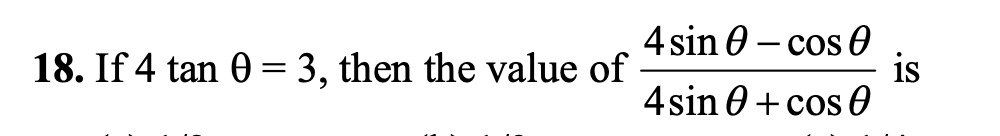(a) 1/2 (b) 1/3 (c) 1/4 (d) 1/5

DIRECTION: In question number 19 and 20, a statement of Assertion (A) is followed by a statement of Reason (R). Choose the correct option

19. Statement A (Assertion): The number 6n never end with digit 0 for any natural number n.. Statement R( Reason): The number 9n never end with digit 0 for any natural number n.

(a) Both assertion (A) and reason (R) are true and reason (R) is the correct explanation of assertion (A)

(b) Both assertion (A) and reason (R) are true and reason (R) is not the correct explanation of assertion (A)
(c) Assertion (A) is true but reason (R) is false.
(d) Assertion (A) is false but reason (R) is true.

19. Statement A (Assertion): The value of y is 6, for which the distance between the points P(2, – 3) and Q(10, y) is 10.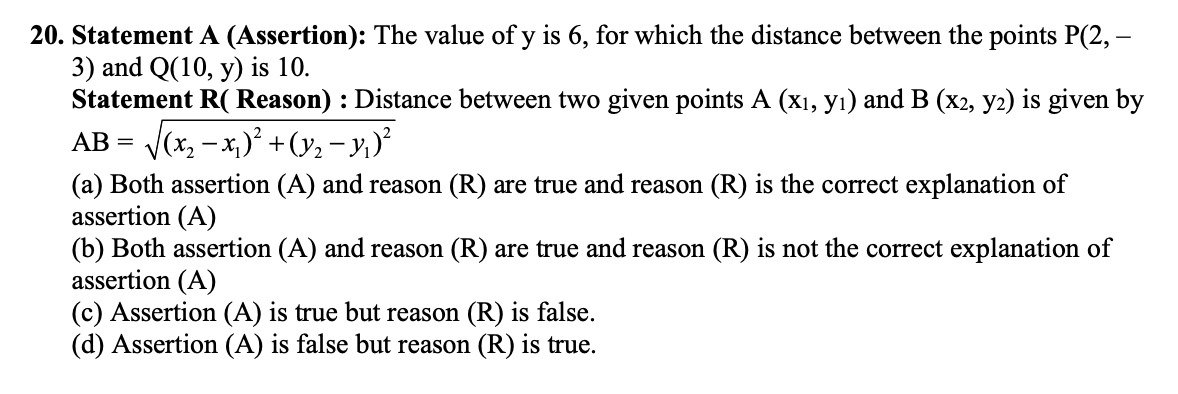Section B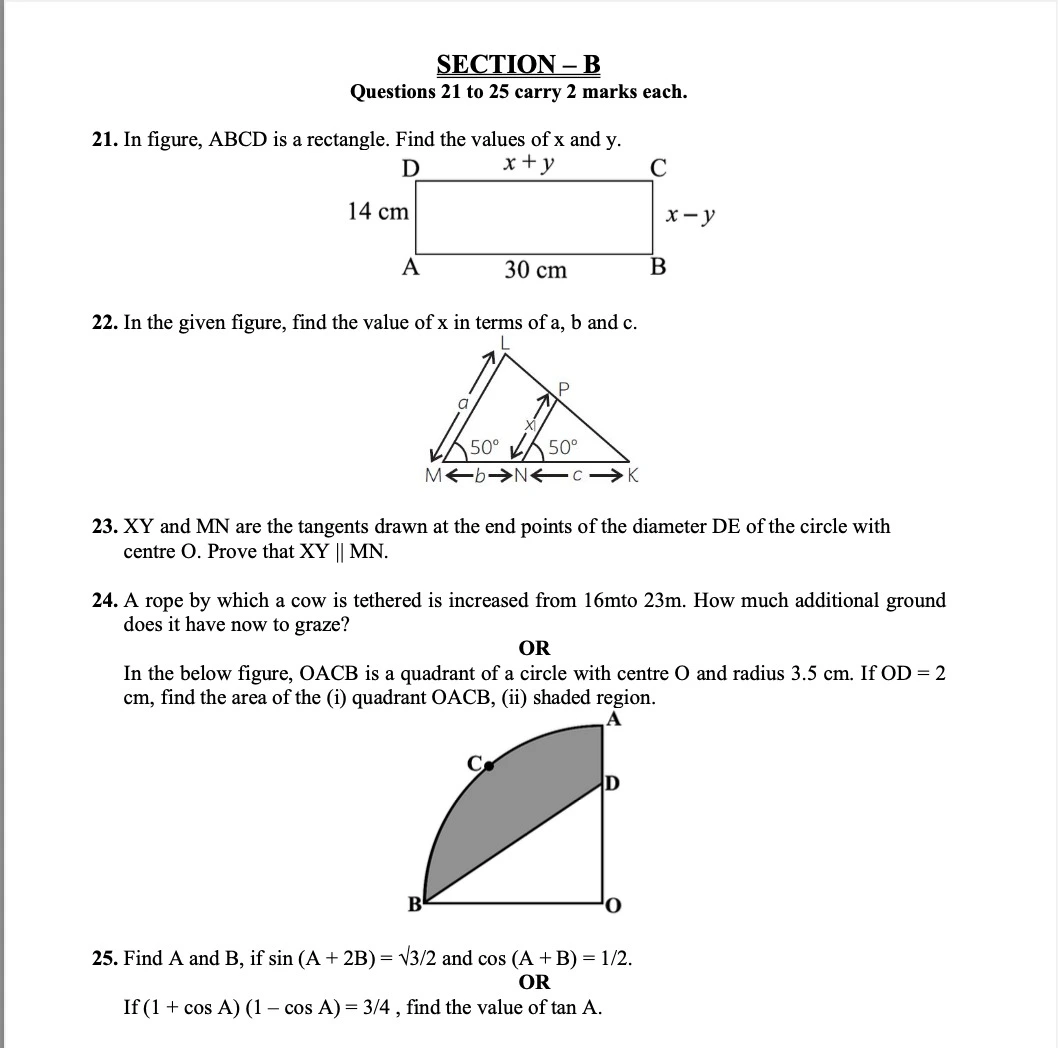#### SECTION – C Questions 13 to 22 carry 3 marks each

26. Prove that √5 is an irrational number.

27. Find the zeroes of the quadratic polynomial 6×2 – 7x – 3 and verify the relationship between the zeroes and the coefficients of the polynomial.

28. A part of monthly hostel charges in a college is fixed and the remaining depends on the number of days one has taken food in the mess. When a student ‘A’ takes food for 22 days, he has to pay Rs. 1380 as hostel charges; whereas a student ‘B’, who takes food for 28 days, pays Rs. 1680 as hostel charges. Find the fixed charges and the cost of food per day.

OR

The ratio of income of two persons is 9 : 7 and the ratio of their expenditure is 4 : 3, if each of them manage to save Rs. 2000/month. Find their monthly incomes.

28. Prove that the intercept of a tangent between two parallel tangents to a circle subtends a right angle at the center

OR

Prove that opposite sides of a quadrilateral circumscribing a circle subtend supplementary angles at the center of the circle.

30. One card is drawn at random from a well-shuffled deck of 52 playing cards. Find the probability that the card drawn is

(i) either a red card or a king, (ii) neither a red card nor a queen.

#### SECTION – D Questions 32 to 35 carry 5 marks each.

31. Two water taps together can fill a tank in 9 3 hours. The tap of a larger diameter takes 10 hours 8 less than the smaller one to fill the tank separately. Find the time at which each tap can separately fill the tank.

OR

A rectangular park is to be designed whose breadth is 3 m less than its length. Its area is to be 4 square meters more than the area of a park that has already been made in the shape of an isosceles triangle with its base as the breadth of the rectangular park and of altitude 12 m. Find its length and breadth.

32. Prove that if a line is drawn parallel to one side of a triangle intersecting the other two sides in distinct points, then the other two sides are divided in the same ratio.
Using the above theorem proves that a line through the point of intersection of the diagonals and parallel to the base of the trapezium divides the nonparallel sides in the same ratio.

33. A vessel is in the form of an inverted cone. Its height is 8 cm and the radius of its top which is open is 5 cm. It is filled with water up to the brim. When lead shots, each of which is a sphere of radius 0.5 cm are dropped into the vessel, one-fourth of the water flows out. Find the number of lead shots dropped into the vessel.

OR

A copper wire of diameter 8 mm is evenly wrapped on a cylinder of length 24 cm and diameter 49 cm to cover the whole surface. Find (i) the length of the wire and (ii) the volume of the wire.

#### SECTION – E(Case Study Based Questions) Questions 35 to 37 carry 4 marks each.

34. In the sport of cricket the Captain sets the field according to a plan. He instructs the players to take a position at a particular place. There are two reasons to set a cricket field—to take wickets and to stop runs being scored.

The following graph shows the position of players during a cricket match.

(i) Find the coordinate of the point on y-axis which are equidistant from the points representing the players at Cover P(2, –5) and Mid-wicket Q(–2, 9)
(ii) Find the ratio in which x-axis divides the line segment joining the points Extra Cover S(3, – 3) and Fine Leg (–2, 7).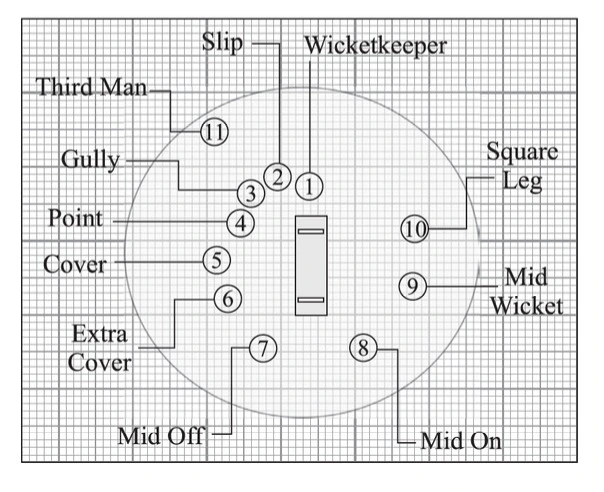35. Anita’s mother start a new shoe shop. To display the shoes, she put 3 pairs of shoes in 1st row, 5 pairs in 2nd row, 7 pairs in 3rd row and so on.On the basis of the above information, answer the following questions.
(i) If she puts a total of 120 pairs of shoes, then find the number of rows required. (ii) What is the difference of pairs of shoes in 17th row and 10th row.

36. A 1.2 m tall girl spots a balloon moving with the wind in a horizontal line at a height of 88.2 m from the ground. The angle of elevation of the balloon from the eyes of the girl at any instant is 60°. After 30 seconds, the angle of elevation reduces to 30° (see the below figure).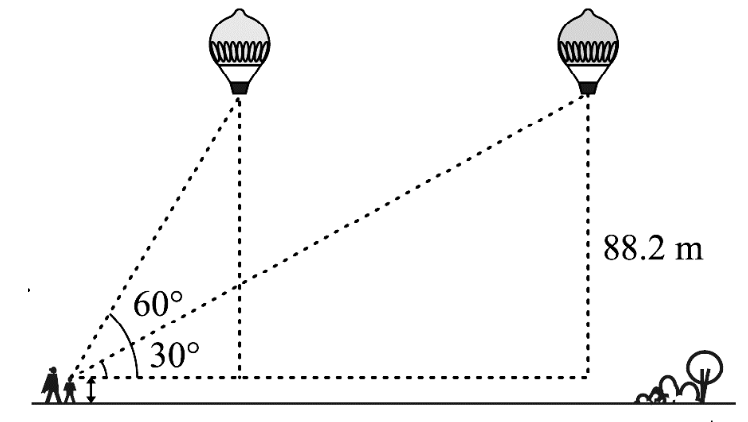Based on the above information, answer the following questions. (Take √3 =1.732)

(i) Find the distance travelled by the balloon during the interval.
(ii) Find the speed of the balloon.

Test Paper Source: KENDRIYA VIDYALAYA GACHIBOWLI, GPRA CAMPUS

CBSE Sample Papers | CBSE Circulars |  Quizzes | Study Material